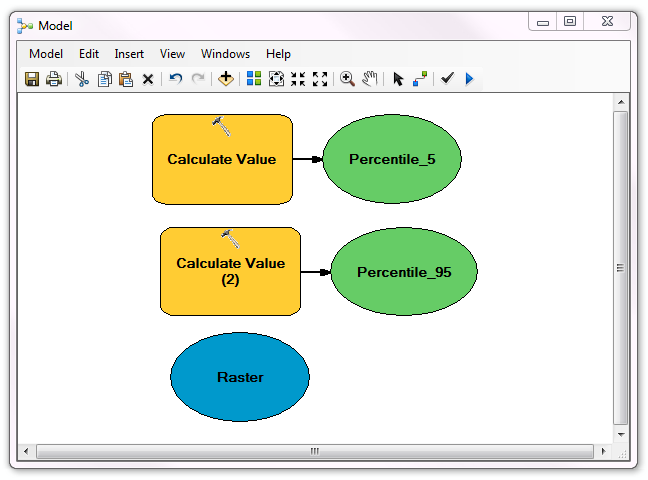# Model builder - automatic selection of quantiles for raster data

3870
4
07-13-2015 02:19 AM
Highlighted

I would like to find values of quantile 5% and quantile 95% of my data. I know that I can find statistics using Get Raster Properties; but there is no possibility to get the quantile values of the data. Finally I have found the quantile values using Reclassify option. I reclassified my data for three classes using Quantile as a method for classification with thresholds: 5, 95, and 100%

However, here I have a problem, because I would like to apply the evaluated quantiles in Model Builder am when I change the input data I have to evaluate the qauntiles every time manually, because model builder is not doing this automatically. Is there some other way to evaluate quantiles which can be fully automatically used in Model Builder?

Thanks in advance for some suggestions.

Tags (4)
4 Replies
Highlighted
byMVP Frequent Contributor

Karolina,

First of all I am not a statistician so when you wrote find values of quantile 5% and quantile 95% of my data I interpreted that as percentiles, so my following example may not be giving you the correct values! What it does show is how you compute values that the standard raster property tools do not return. By using the approach below you can compute the values you need and integrate them into model builder.

Below is a model with two Model only tools Calculate Value returning the 5% and 95% percentile of a raster.If for example,I open the 95% percentile tool you can see the python code used to compute the value. The trick is to turn it into a numpy array and then you have access to many of the array processing functions.Dan Patterson has written many guides doing very clever stuff with numpy arrays, too clever for me!

Highlighted

I have been attempting the above with no success. A value of zero is returned for any percentiles below 86%. Are there any requirements/preconditions for the raster data set?

Highlighted

I believe I have sourced the issue. How does one exclude the NoData values?

Highlighted
byMVP Esteemed Contributor

NoData values should be converted to None, according to the doc.  I'd try this with a small dataset at the python prompt and look at the array on the numpy side to see if things are what you think they are.

Quantile breaks can be unexpected when there are a lot ties (many cells with the same value) in your data.